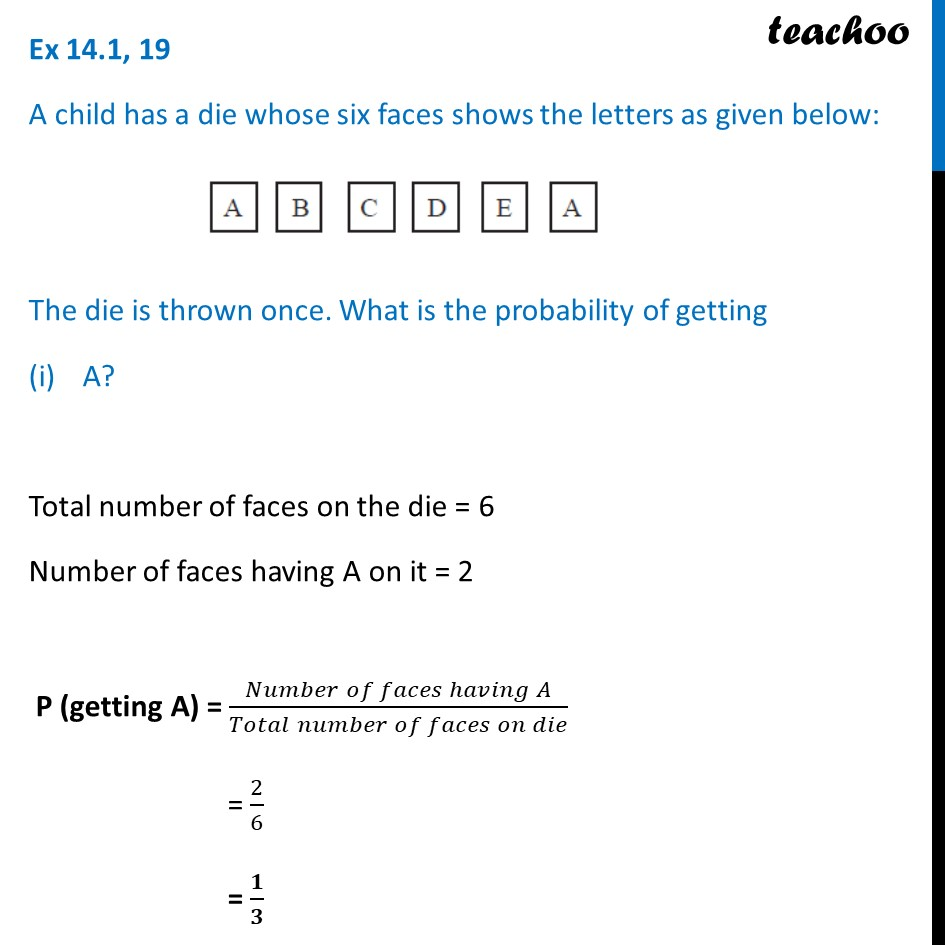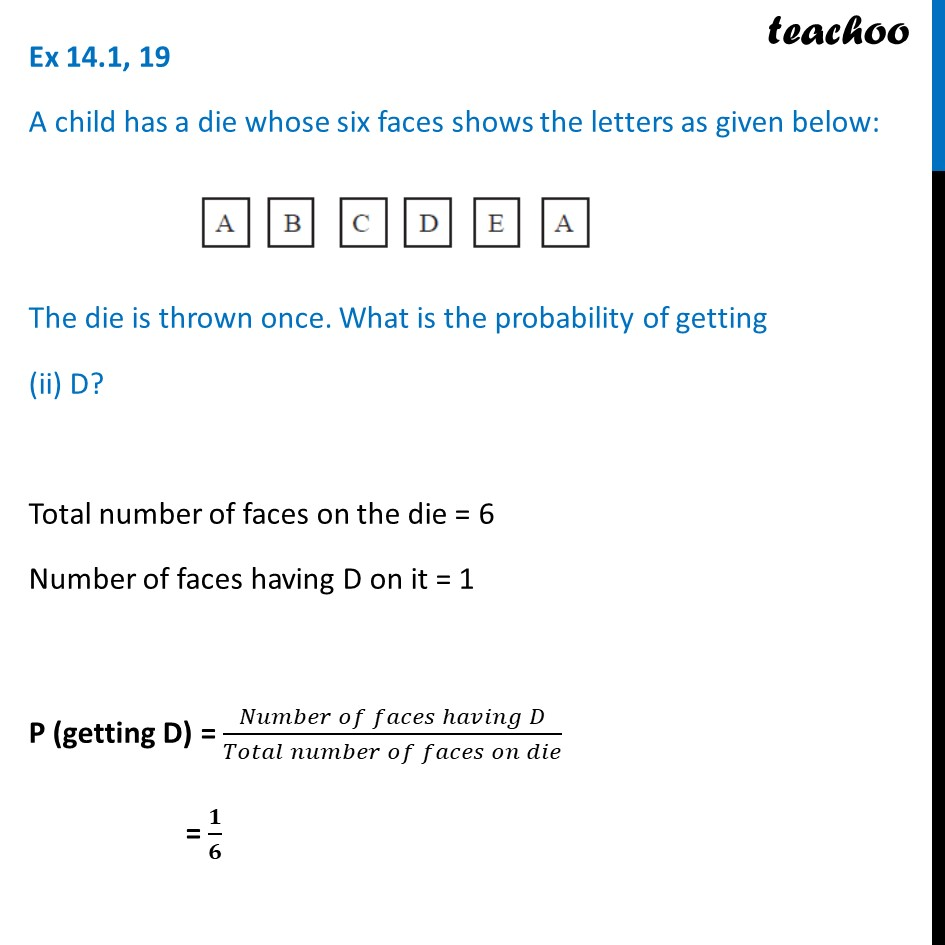Chapter 15 Class 10 Probability

Class 10
Important Questions for Exam - Class 10Learn in your speed, with individual attention - Teachoo Maths 1-on-1 Class

### Transcript

Ex 14.1, 19 A child has a die whose six faces shows the letters as given below: The die is thrown once. What is the probability of getting A? Total number of faces on the die = 6 Number of faces having A on it = 2 P (getting A) = (𝑁𝑢𝑚𝑏𝑒𝑟 𝑜𝑓 𝑓𝑎𝑐𝑒𝑠 ℎ𝑎𝑣𝑖𝑛𝑔 𝐴)/(𝑇𝑜𝑡𝑎𝑙 𝑛𝑢𝑚𝑏𝑒𝑟 𝑜𝑓 𝑓𝑎𝑐𝑒𝑠 𝑜𝑛 𝑑𝑖𝑒) = 2/6 = 𝟏/𝟑 Ex 14.1, 19 A child has a die whose six faces shows the letters as given below: The die is thrown once. What is the probability of getting (ii) D? Total number of faces on the die = 6 Number of faces having D on it = 1 P (getting D) = (𝑁𝑢𝑚𝑏𝑒𝑟 𝑜𝑓 𝑓𝑎𝑐𝑒𝑠 ℎ𝑎𝑣𝑖𝑛𝑔 𝐷)/(𝑇𝑜𝑡𝑎𝑙 𝑛𝑢𝑚𝑏𝑒𝑟 𝑜𝑓 𝑓𝑎𝑐𝑒𝑠 𝑜𝑛 𝑑𝑖𝑒) = 𝟏/𝟔